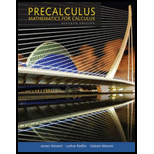Chapter 13.3, Problem 36E### Precalculus: Mathematics for Calcu...

7th Edition
James Stewart + 2 others
ISBN: 9781305071759

#### Solutions

Chapter
Section### Precalculus: Mathematics for Calcu...

7th Edition
James Stewart + 2 others
ISBN: 9781305071759
Textbook Problem

# Which Derivative Does the Limit Represent? The given limit represents the derivative of a function f at a number a. Find f and a.36. lim h → 0 cos ( π + h ) + 1 h

To determine

To evaluate: The function f and the number a in the given limit derivative of a function.

Explanation

Given:

The limit derivative of the function is given as:

limh0cos(π+h)+1h

The derivative of the function at the point P(a,f(a)) is represented as:

f(a)=limh0f(a+h)f(a)h at x=a , (1)

Calculation:

Now, compare the given limit derivative of the function with equation (1).

limh0f(a+h)f(a)h=limh0cos(π+h)+1h

It can be written as:

limh0f(a+h)f(a)h=limh0cos(π+h)(1)h

In the given problem, cos(π+h) substituted for f(a+h) .

Now, compare these substituted values,

f(a+h)=cos(π+h)

Since, it is clear that the a+h is the increment in the function of x at a number a .

So,

x=a+h

Here,

a+h=π+h

Then,

x=π+h

So, the place the variable x in place of π+h

### Still sussing out bartleby?

Check out a sample textbook solution.

See a sample solution

#### The Solution to Your Study Problems

Bartleby provides explanations to thousands of textbook problems written by our experts, many with advanced degrees!

Get Started

## Additional Math Solutions

#### Find more solutions based on key concepts